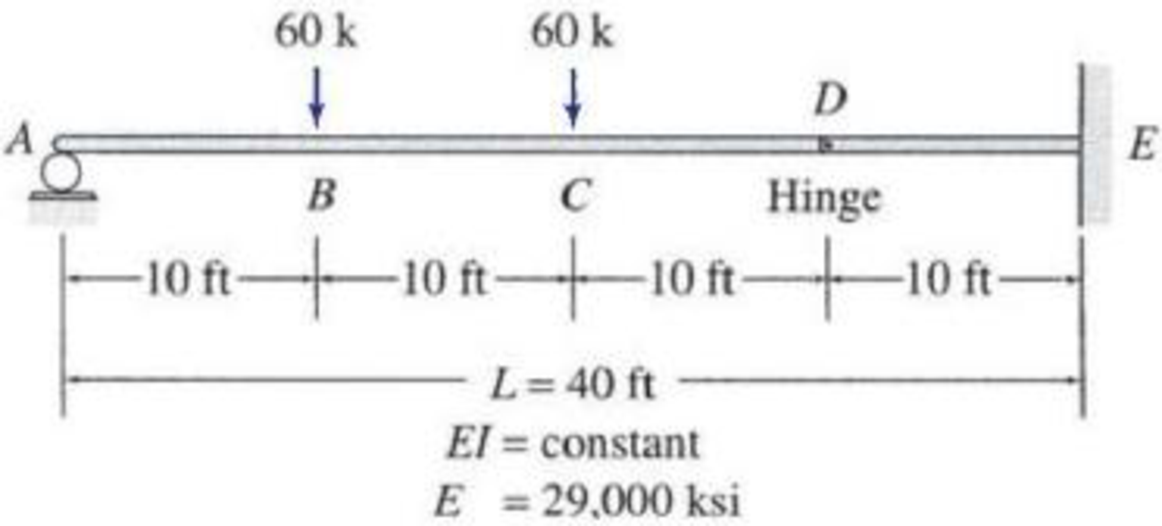# 6.18 through 6.22 Determine the smallest moment of inertia I required for the beam shown, so that its maximum deflection does not exceed the limit of 1/360 of the span length (i.e., Δ max ≤ L /360). Use the moment-area method. FIG. P6.22, P6.48

#### Solutions

Chapter
Section
Chapter 6, Problem 22P
Textbook Problem
434 views

## 6.18 through 6.22 Determine the smallest moment of inertia I required for the beam shown, so that its maximum deflection does not exceed the limit of 1/360 of the span length (i.e., Δmax ≤ L/360). Use the moment-area method.FIG. P6.22, P6.48To determine

Find the smallest moment of inertia (I) required for the beam if the deflection does not exceed the limit l/360 of the span length by using moment-area method.

### Explanation of Solution

Given information:

The Young’s modulus (E) is 29,000 ksi.

Calculation:

Consider the flexural rigidity EI of the beam is constant.

Show the given beam as in Figure (1).

Refer Figure (1),

Consider upward is positive and downward is negative.

Consider clockwise is negative and counterclockwise is positive.

Determine the support reaction at A using the Equation of equilibrium;

ME=0RA×40+(60×30)+(60×20)600=0RA=2,40040RA=60k

Determine the reaction at support E;

V=0RA+RE6060=0RE=12060RE=60k

Show the reactions of the given beam as in Figure (2).

Refer Figure (2),

Determine the bending moment at E;

ME=(60×40)(60×30)(60×20)=2,4003,000=600kips-ft

Determine the bending moment at D;

MD=600(60×10)=600600=0

Determine the bending moment at C;

MC=(60×20)(60×10)=1,200600=600kips-ft

Determine the bending moment at B;

MB=(60×10)=600kips-ft

Determine the bending moment at A;

MA=(60×40)600(60×20)(60×10)=2,4002,400=0

Show the M/EI diagram of the given beam as in Figure (3).

Show the deflected shape of the given beam as in Figure (4).

Determine the deflection between A and D using the relation;

Substitute 600EI for MEI, (12×10×(13×10+20)) for area of triangle, (10×(102+10)) for area of rectangle, and (12×10×(23×10)) for area of triangle.

ΔDA=[600EI(12×10×(13×10+20))+(600EI)(10×(102+10))+600EI(12×10×(23×10))]=1EI[70,000+90,000+20,000]=180,000kipsft3EI

Determine the deflection between D and E using the relation;

ΔDE=[MEI(Areaoftriangle)]

Substitute 600EI for MEI and (12×10×(23×10)) for area of triangle

### Still sussing out bartleby?

Check out a sample textbook solution.

See a sample solution

#### The Solution to Your Study Problems

Bartleby provides explanations to thousands of textbook problems written by our experts, many with advanced degrees!

Get Started

Find more solutions based on key concepts
Describe the active solar heating systems. How do they function?

Engineering Fundamentals: An Introduction to Engineering (MindTap Course List)

What is a surrogate key, and when should you use one?

Database Systems: Design, Implementation, & Management

What is a recursive join?

Database Systems: Design, Implementation, & Management

What hardness testing scale uses many different penetrators and loads?

Precision Machining Technology (MindTap Course List)

What is an InfoSec blueprint?

Management Of Information Security

Define and identify issues surrounding content and web filtering.

Enhanced Discovering Computers 2017 (Shelly Cashman Series) (MindTap Course List)

If your motherboard supports ECC DDR3 memory, can you substitute non-ECC DDR3 memory?

A+ Guide to Hardware (Standalone Book) (MindTap Course List)

List some applications that use high-pressure piping.

Welding: Principles and Applications (MindTap Course List)